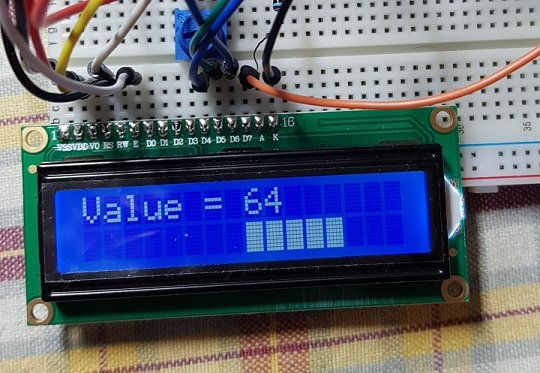# B4R LibraryrLcdBarGraph - draw a bar graph on your LCD display (LCD 1602)

A wrap for this Github project. Attached a B4R sample project and the library. Extract the library from rLcdBarGraph.zip and copy the folder and its contents to your B4R additional libs folder. Copy the xml to the root of your B4R additional libs folder.

Sure you will figure out numcolumns, startX, and startY (there is pop up help in the library)

Wiring diagram:What it looks like (in the attached example I am starting the bar graph in row 1, column 8 and are using 8 columns (numcolumns) for the bar graph)Sample Code:
B4X:
``````#Region Project Attributes
#AutoFlushLogs: True
#CheckArrayBounds: True
#StackBufferSize: 300
#End Region

Sub Process_Globals
Public Serial1 As Serial
Private lcd As LiquidCrystal        'create instance of rLiquidCrystal library
Private lcdbg As LcdBarGraph        'create instance of rLcdBarGraph library

Dim numcolumns As Byte = 8          'number of columns to use for displaying the bar graph
Dim startX As Byte = 8              'start displaying the bar graph at Column 8 (first column is column 0) of LCD1602
Dim startY As Byte = 1              'start displaying the bar graph on the second row (first row is 0) of LCD1602

Dim t As Timer

End Sub

Private Sub AppStart
Serial1.Initialize(115200)
t.Initialize("t_tick", 1000)

' Init the LCD: RS,RW,EN,Data(4)
lcd.Initialize(8,255,9, Array As Byte(4,5,6,7))
lcd.Begin(16,2)
lcd.DisplayOn = True
' Init the bignum with lcd display
lcd.Clear
'initialize LcdBarGraph with the number of columns to use, the column start position (0 to 16 for LCD1602), and the row start position (0 or 1 for LCD1602)
lcdbg.Initialize(lcd, numcolumns, startX, startY)
t.Enabled = True

End Sub

Sub t_tick
lcd.SetCursor(0, 0)
lcd.Write("Value = ")
Dim mynum As Int = Rnd(0, 101)
lcd.SetCursor(8, 0)
lcd.Write(" ")
lcd.Write(" ")
lcd.Write(" ")
lcd.SetCursor(8, 0)
lcd.Write(mynum)
lcdbg.drawValue(mynum, 100)

End Sub``````

#### Attachments

• b4rLcdBarGraph.zip
1.1 KB · Views: 508
• rLcdBarGraph.zip
5.1 KB · Views: 524